# 图上嵌图片

R 基础图形库（base graphics）可以在做图的时候嵌入图片，使用的是graphics::rasterImage

imgurl <- "http://phylopic.org/assets/images/submissions/295cd9f7-eef2-441e-ba7e-40c772ca7611.256.png"
library(EBImage)
plot(1, type = "n", xlab = "", ylab = "", xlim = c(0, 8), ylim = c(0, 8))
rasterImage(x, 2, 2, 6, 4)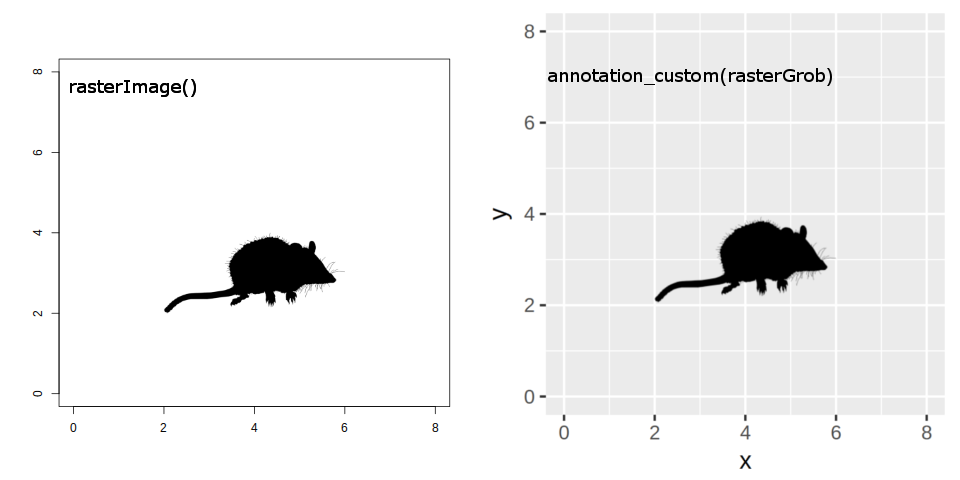library(grid)
library(ggplot2)

p <- ggplot(d = data.frame(x = c(0, 8), y = c(0, 8)), aes(x, y)) + geom_blank()
p + annotation_custom(rasterGrob(x), 2, 6, 2, 4)


ggimage就是来实现这样一个功能，它只是一个简单的包，允许我们在ggplot2中把离散性变量映射到不同的图片来画图。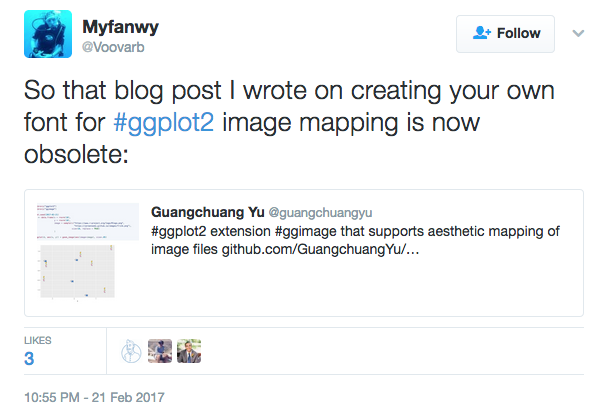ggtree和可视化有关的函数分两类，一类是加注释的图层，另一类是可视化操作树（比如像旋转、合并分支）。操作树的都是普通函数，而加注释的都是geom图层，除了subviewphylopic，这种所谓逼死处女座的存在，我早就想改成了geom_subviewgeom_phylopic了（已实现），这也是为什么我要写ggimage的原因了。

# 安装

ggimage依赖于EBImage来读图片，这是个Bioconductor包，所以我们需要额外的动作来安装它，用setRepositories把Bioconductor软件仓库加进来，这样install.packages也可以搜索到它的包。

setRepositories(ind = 1:2)
install.packages("ggimage")


# 实例分析

ggflags这几个，都是为特定的目的而实现的，都有其特定的应用场景，而ggimage是的
geom_image是通用的，通过对它进行简单的包装，同样可以实现这些特殊场景的应用图层。

CatterPlots这个包只可以应用于基础图形库（base graphics）中，通过预设的几个猫图（R对象，随包载入）来画散点图。最近RevolutionAnalytics 有博文介绍。ggplot2没有相应画猫的包。我们可以使用ggimage来画，而且不用限制于CatterPlots预设的几个图形。

library(ggplot2)
library(ggimage)

mytheme <- theme_minimal() +
theme(axis.title = element_blank())
theme_set(mytheme)

x <- seq(-2 * pi, 2 * pi, length.out = 30)
d <- data.frame(x = x, y = sin(x))

img <- "http://www.belleamibengals.com/bengal_cat_2.png"
ggplot(d, aes(x, y)) + geom_image(image = img, size = .1)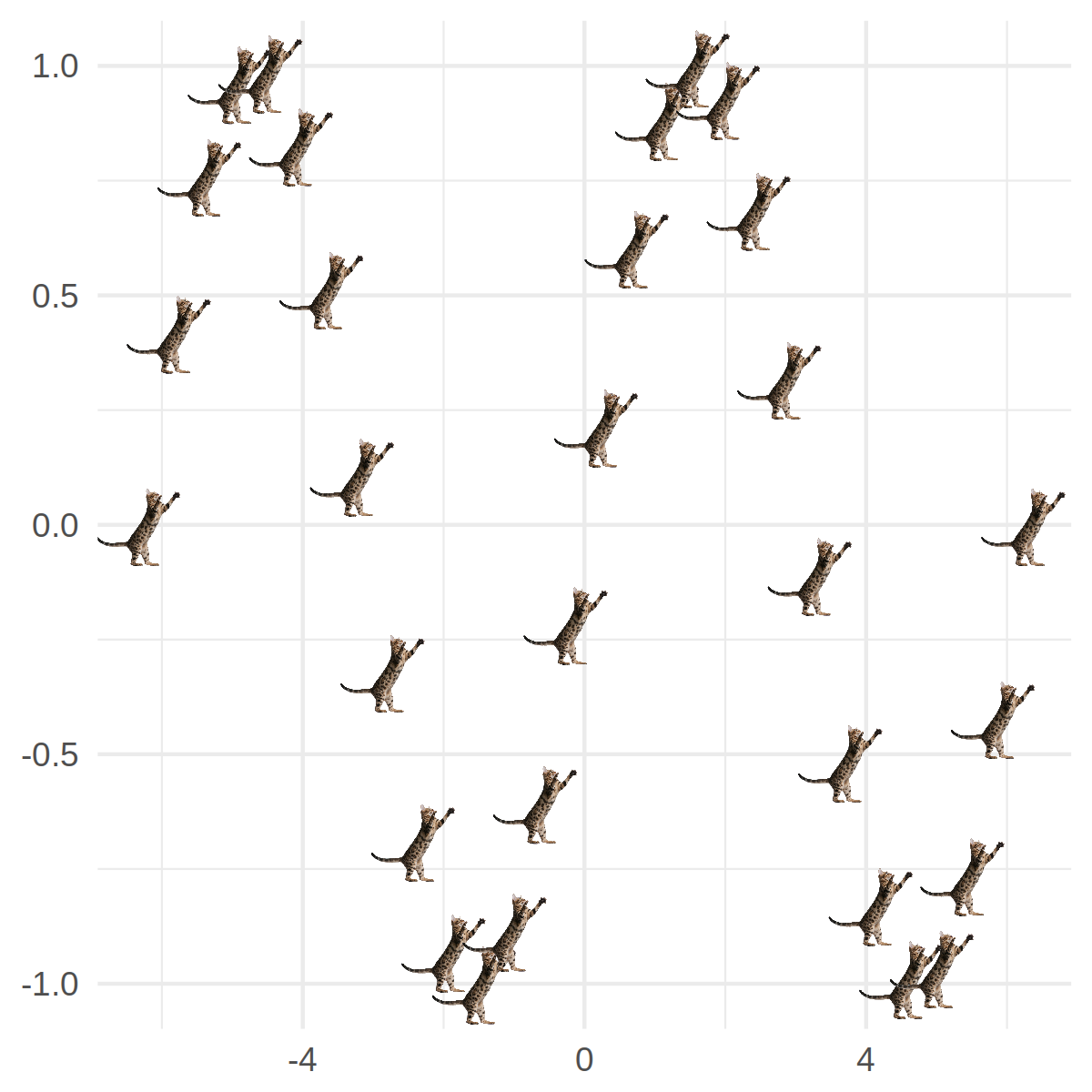CatterPlots实现的方式就是上面谈到的rasterImage内部使用了循环。rphylopic同时支持基础图形库（base graphics）和ggplot2，也是一样的实现方式，不过rphylopic内部没有使用循环，一次只能加一个图，它使用的图来自于phylopic数据库。

ggplot(d, aes(x, y)) + geom_phylopic(image = "500bd7c6-71c1-4b86-8e54-55f72ad1beca", size = .1)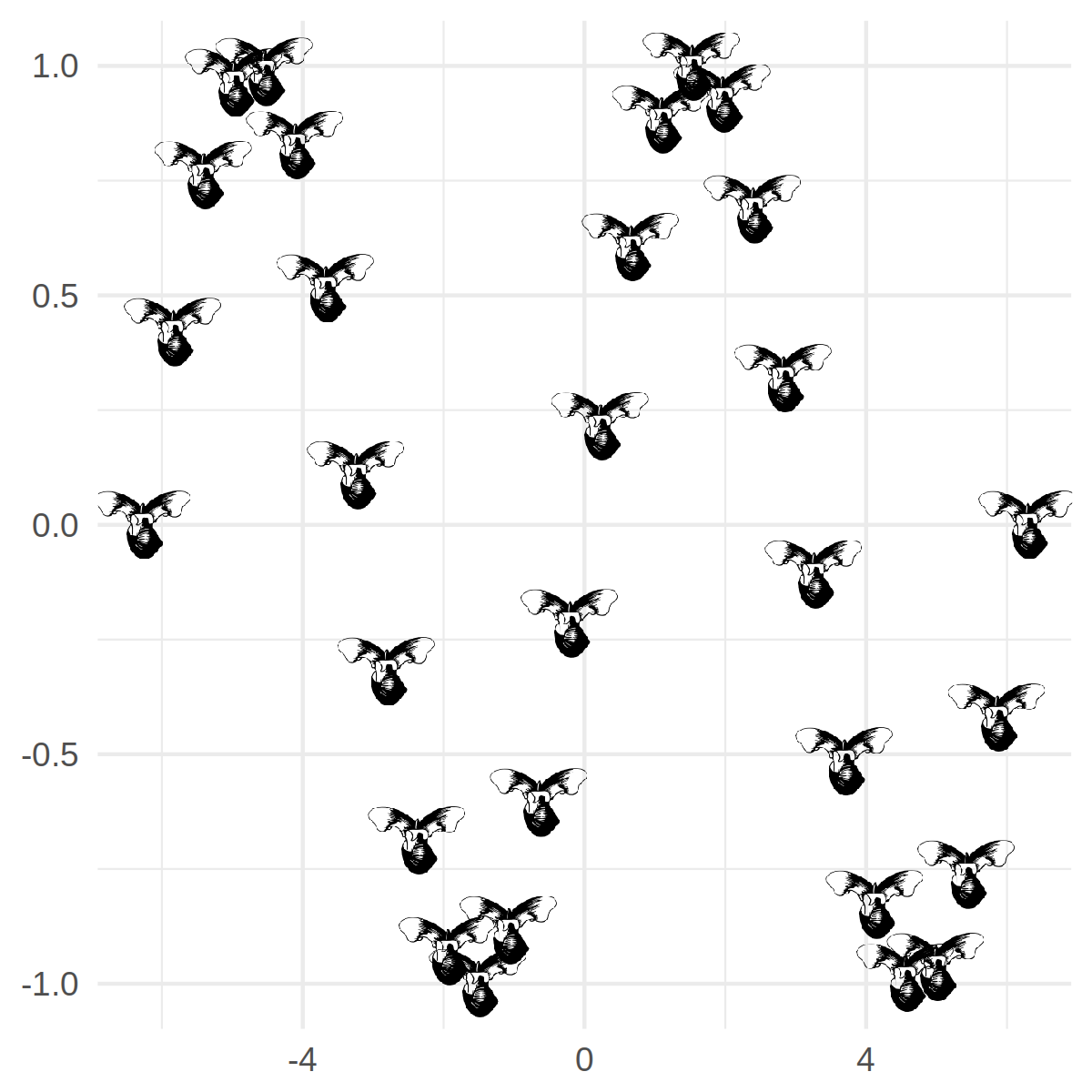emoGG是专门来画emoji的，如果要画emoji的话，我推荐我写的emojifont包，在轩哥的showtext基础上，把emoji当做普通字体一样操作，更方便。

emoGG这个包提供了geom_emoji图层，虽然一次可以画出散点，但不支持aes映射，而ggimage所提供的geom_emoji则支持映射，下面的例子中我们做了一个简单的回归分析，如果残差<0.5用笑脸表示，>0.5则用哭脸来表示。

set.seed(123)
iris2 <- iris[sample(1:nrow(iris), 30), ]
model <- lm(Petal.Length ~ Sepal.Length, data = iris2)
iris2$fitted <- predict(model) p <- ggplot(iris2, aes(x = Sepal.Length, y = Petal.Length)) + geom_linerange(aes(ymin = fitted, ymax = Petal.Length), colour = "purple") + geom_abline(intercept = model$coefficients,
slope = model$coefficients) p + ggimage::geom_emoji(aes(image = ifelse(abs(Petal.Length-fitted) > 0.5, '1f622', '1f600')))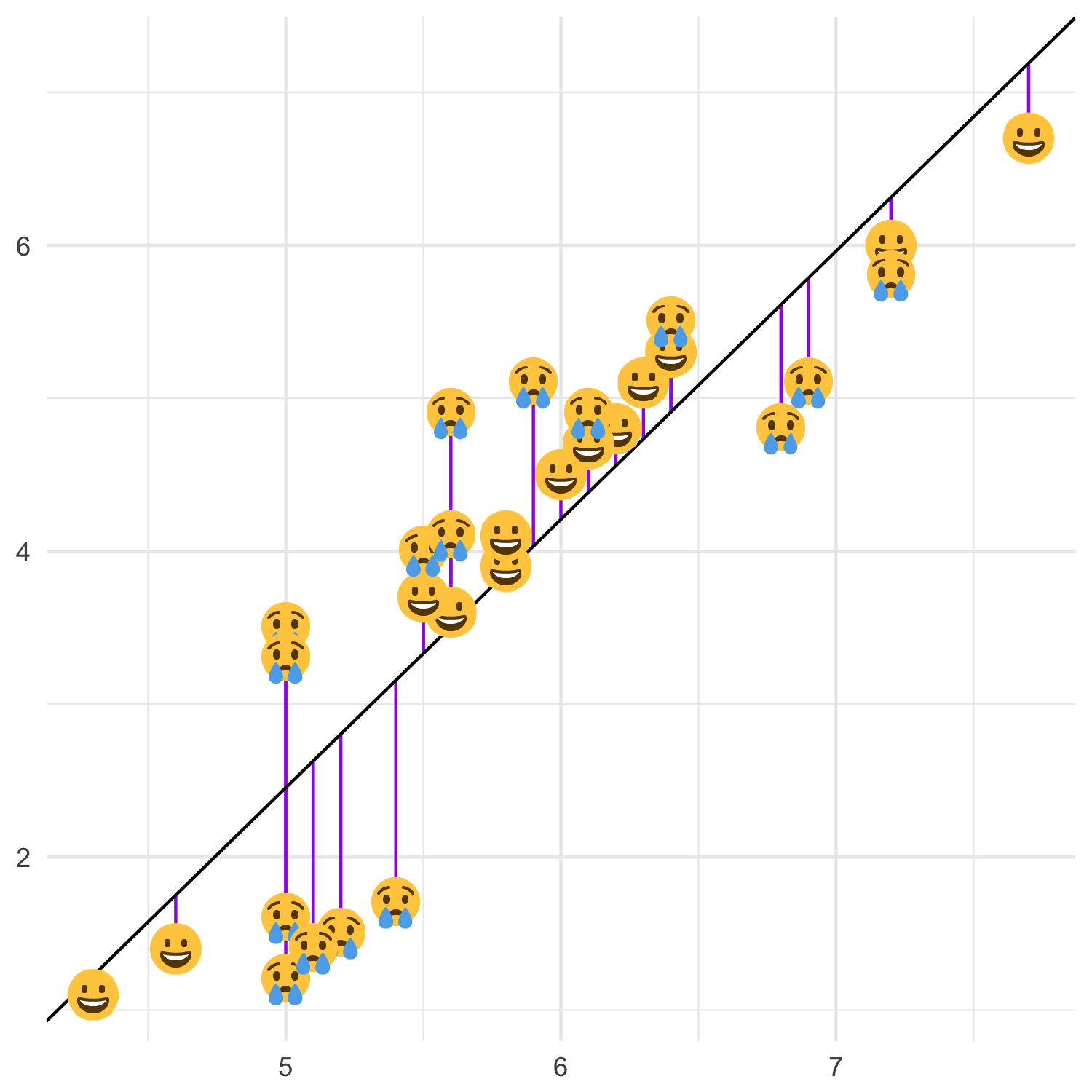如果要用emoGG来做的话，则需要自己切数据分两次来进行： p + emoGG::geom_emoji(data = subset(iris2, (Petal.Length-fitted) < 0.5), emoji = "1f600") + emoGG::geom_emoji(data = subset(iris2, (Petal.Length-fitted) > 0.5), emoji = "1f622")  这里我们只分两类(残差是否大于0.5)，所以需要加两次，试想我们的分类变量有多种可能的取值，则我们需要分多次切数据加图层，CatterPlotsrphylopicemoGG都有这个问题，这也是aes映射之于ggplot2的重要和强大之处，它让我们可以在更高的抽像水平思考， ggflags是支持aes映射的，只不过它只能用来画国旗而已。同样ggimage也提供 了相应的geom_flag来使用国旗用于做图。 library(rvest) library(dplyr) url <- "http://www.nbcolympics.com/medals" medals <- read_html(url) %>% html_nodes("table") %>% html_table() %>% .[] library(countrycode) library(tidyr) medals <- medals %>% mutate(code = countrycode(Country, "country.name", "iso2c")) %>% gather(medal, count, Gold:Bronze) %>% filter(Total >= 10) head(medals)  Country Total code medal count Russia 33 RU Gold 13 United States 28 US Gold 9 Norway 26 NO Gold 11 Canada 25 CA Gold 10 Netherlands 24 NL Gold 8 Germany 19 DE Gold 8 首先我们从网站上爬回来2016年各个国家的奥林匹克奖牌数，画出柱状图，并在xlab国家名边上用ggimage画上国旗： p <- ggplot(medals, aes(Country, count)) + geom_col(aes(fill = medal), width = .8) p + geom_flag(y = -2, aes(image = code)) + coord_flip() + expand_limits(y = -2) + scale_fill_manual(values = c("Gold" = "gold", "Bronze" = "#cd7f32", "Silver" = "#C0C0C0"))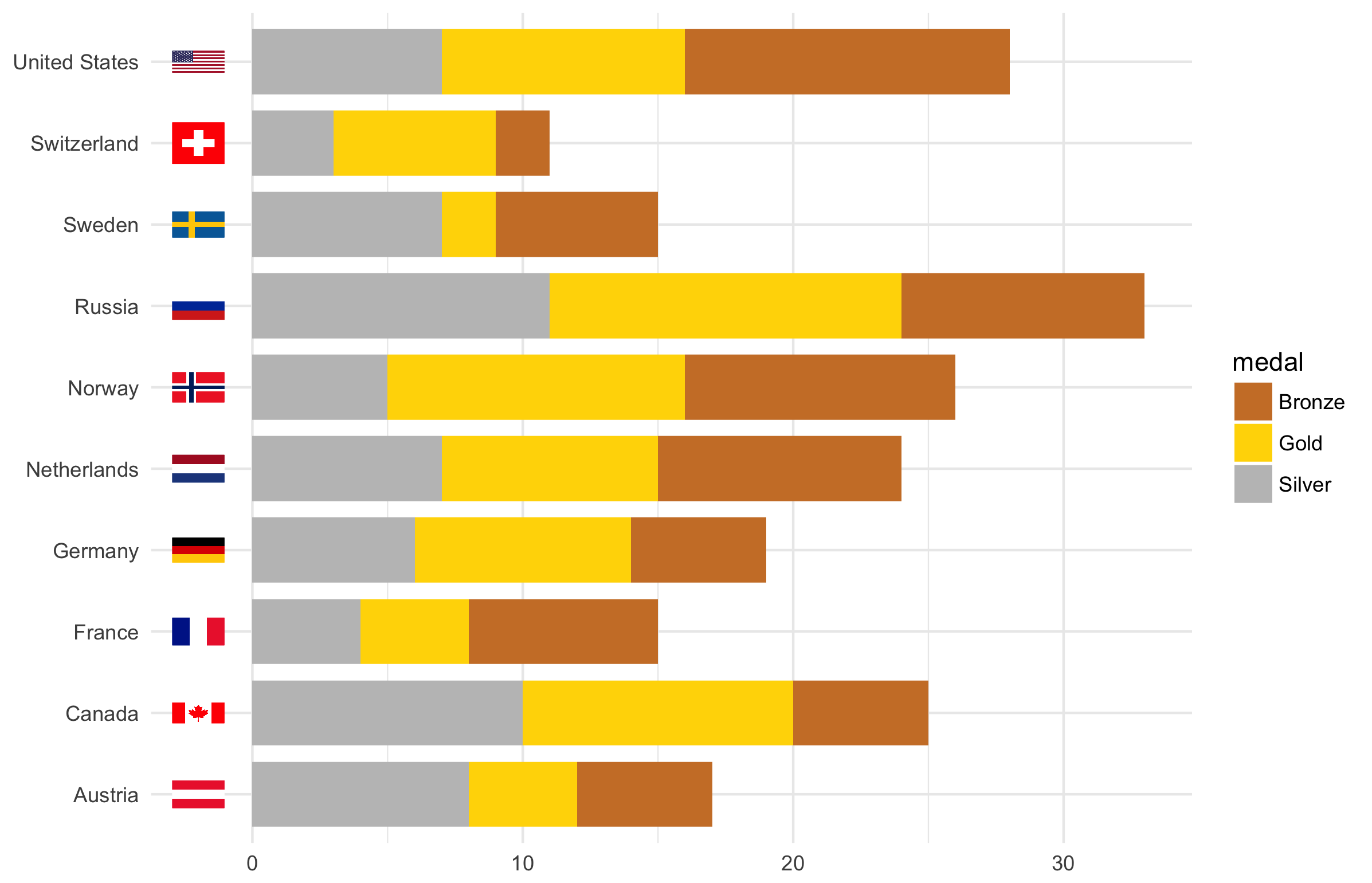## ggimage 前面我们介绍了ggimage在一些场景的应用实例，虽然有专门的包针对这些应用场景，但ggimage在这些领域中的表现要比大多数的包要好（支持aes映射）。但ggimage的使用并不限于这些(geom_phylopicgeom_emojigeom_flag只是通用图层geom_image的简单封装)，这里将展示一些有趣的例子。 ### 用R图标来画R形状 x <- c(2, 2, 2, 2, 2, 3, 3, 3.5, 3.5, 4) y <- c(2, 3, 4, 5, 6, 4, 6, 3, 5, 2) d <- data.frame(x = x, y = y) img <- system.file("img", "Rlogo.png", package = "png") ggplot(d, aes(x, y)) + geom_image(image = img, size = .1) + xlim(0, 6) + ylim(0, 7)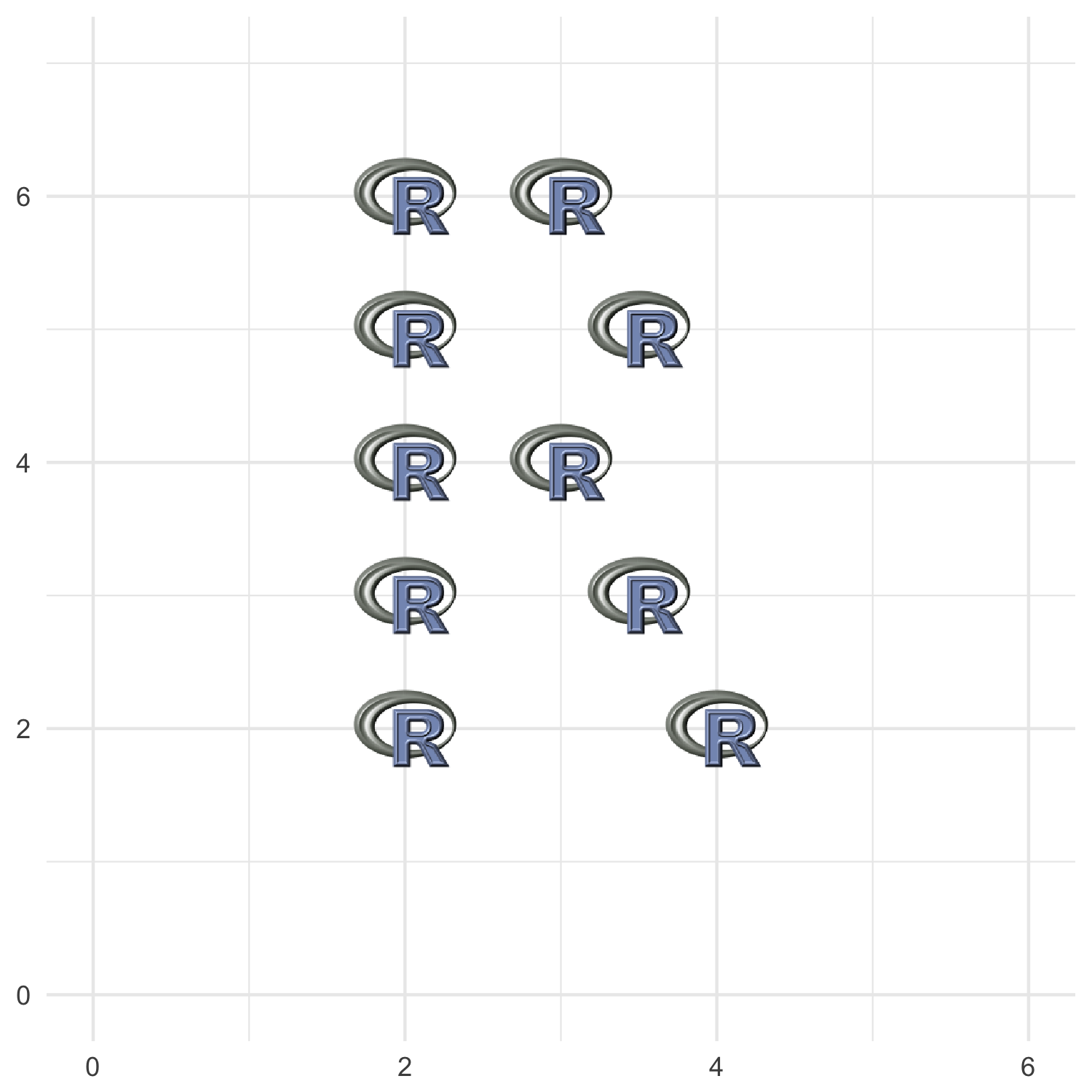### 嵌套式绘图 这里我要展示的是非常有名的气泡图（Bubble Plot），但气泡不是圆圈，而是使用 ggplot2画的饼图，我先把饼图保存起来，再用ggimage拿来画，饼图的大小 与人口总数正相关。这个例子可以应用到很多场景中去，比如一个时间序列的曲线，你要用统计图在某些时间点上展示相关的信息，比如你要在地图上加某些地方的相关统计信息（如果要在地图上画饼图，可以使用我写的scatterpie包）。 crime <- read.csv("http://datasets.flowingdata.com/crimeRatesByState2005.tsv", header = TRUE, sep = "\t", stringsAsFactors = F)  state murder Forcible_rate Robbery aggravated_assult burglary larceny_theft motorvehicletheft population Alabama 8.2 34.3 141.4 247.8 953.8 2650.0 288.3 4627851 Alaska 4.8 81.1 80.9 465.1 622.5 2599.1 391.0 686293 Arizona 7.5 33.8 144.4 327.4 948.4 2965.2 924.4 6500180 Arkansas 6.7 42.9 91.1 386.8 1084.6 2711.2 262.1 2855390 California 6.9 26.0 176.1 317.3 693.3 1916.5 712.8 36756666 Colorado 3.7 43.4 84.6 264.7 744.8 2735.2 559.5 4861515 library(gtable) plot_pie <- function(i) { df <- gather(crime[i, ], type, value, murder:motor_vehicle_theft) ggplot(df, aes(x = 1, value, fill = type)) + geom_col() + coord_polar(theta = 'y') + ggtitle(crime[i, "state"]) + theme_void() + theme_transparent() + theme(legend.position = "none", plot.title = element_text(size = rel(6), hjust = 0.5)) } pies <- sapply(1:nrow(crime), function(i) { outfile <- paste0("crime_", i, ".png") plot_pie(i) + ggsave(outfile, bg = "transparent") outfile }) radius <- sqrt(crime$population / pi)
crime$radius <- 0.2 * radius/max(radius) crime$pie <- pies

leg1 <- gtable_filter(
ggplot_gtable(
ggplot_build(plot_pie(1) + theme(legend.position = "right"))
), "guide-box")

ggplot(crime, aes(murder, Robbery)) +
geom_image(aes(image = pie, size = I(radius))) +
geom_subview(leg1, x = 8.8, y = 50)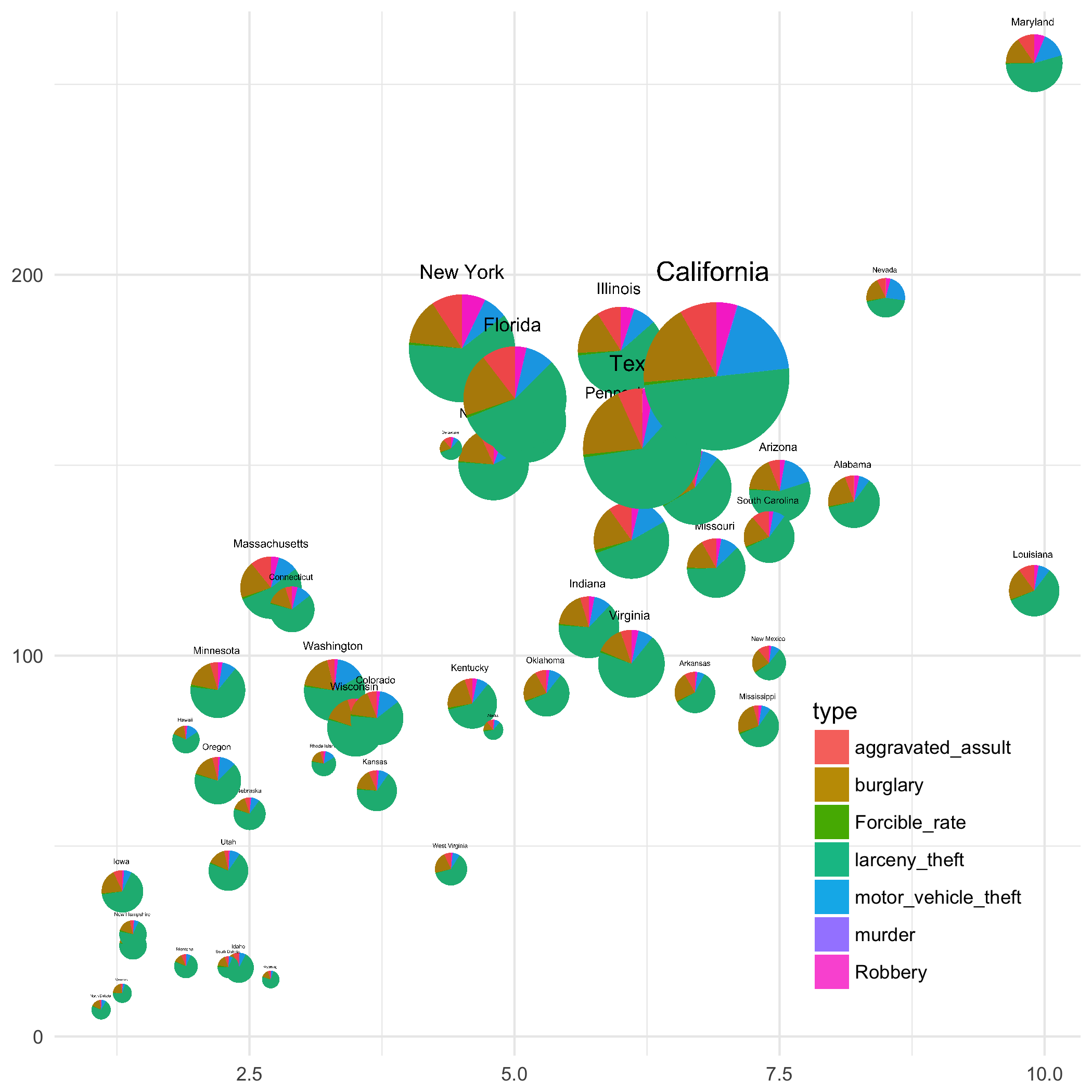plot_crime <- function(i) {
o <- paste0(i, ".png")
ggplot(crime, aes(murder, Robbery)) + geom_blank() +
geom_image(data = crime[i, ], aes(image = pie, size = I(radius))) +
geom_subview(p, leg1, x = 8.8, y = 50) + ggsave(o)
o
}

library(magick)
library(purrr)
order(crime\$murder, decreasing = F) %>%
map(plot_crime) %>%
image_join() %>%
image_animate(fps = 2) %>%
image_write("crime.gif")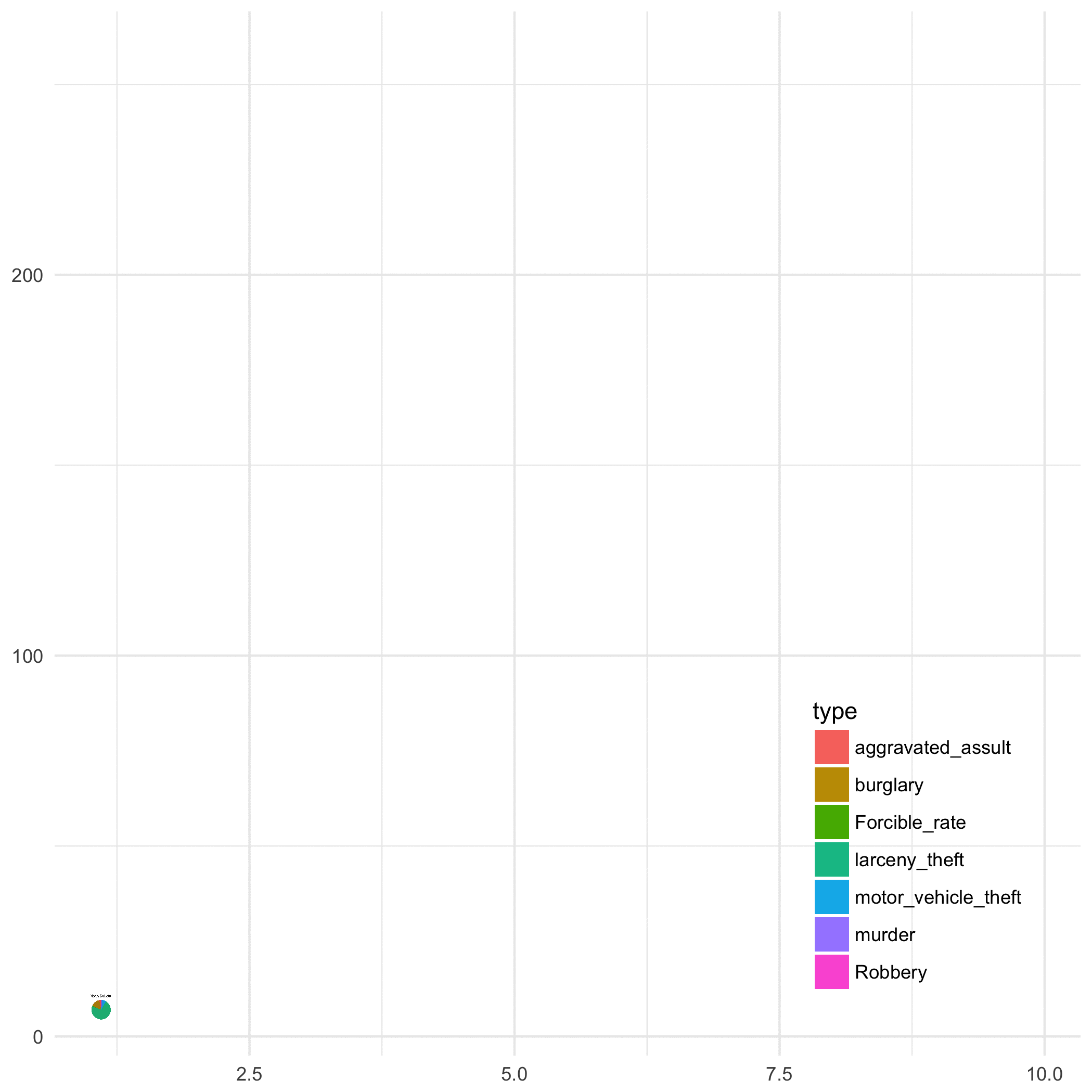geom_subview可以图上嵌图，并不需要保存为图片，但对于ggplot2来讲，保存图片也是有好处的，因为ggplot2画图，点线是在数据空间上，随着我们保存图片的大小是按比例缩小或放大的，但文字是在像素空间上，和画图空间并不相关。所以当我们嵌图时缩小了画图窗口之后，字体会显得格外大，微调起来也比较繁琐，这时候保存为合适尺寸的图片，再用geom_image来加上去，显然就轻松得多。

### 其它来自R社区的例子

SAS博客对M&M巧克力的颜色分布做了分析，通过模拟估计不同颜色的置信区间。这个分析被翻译成R，并产生下图：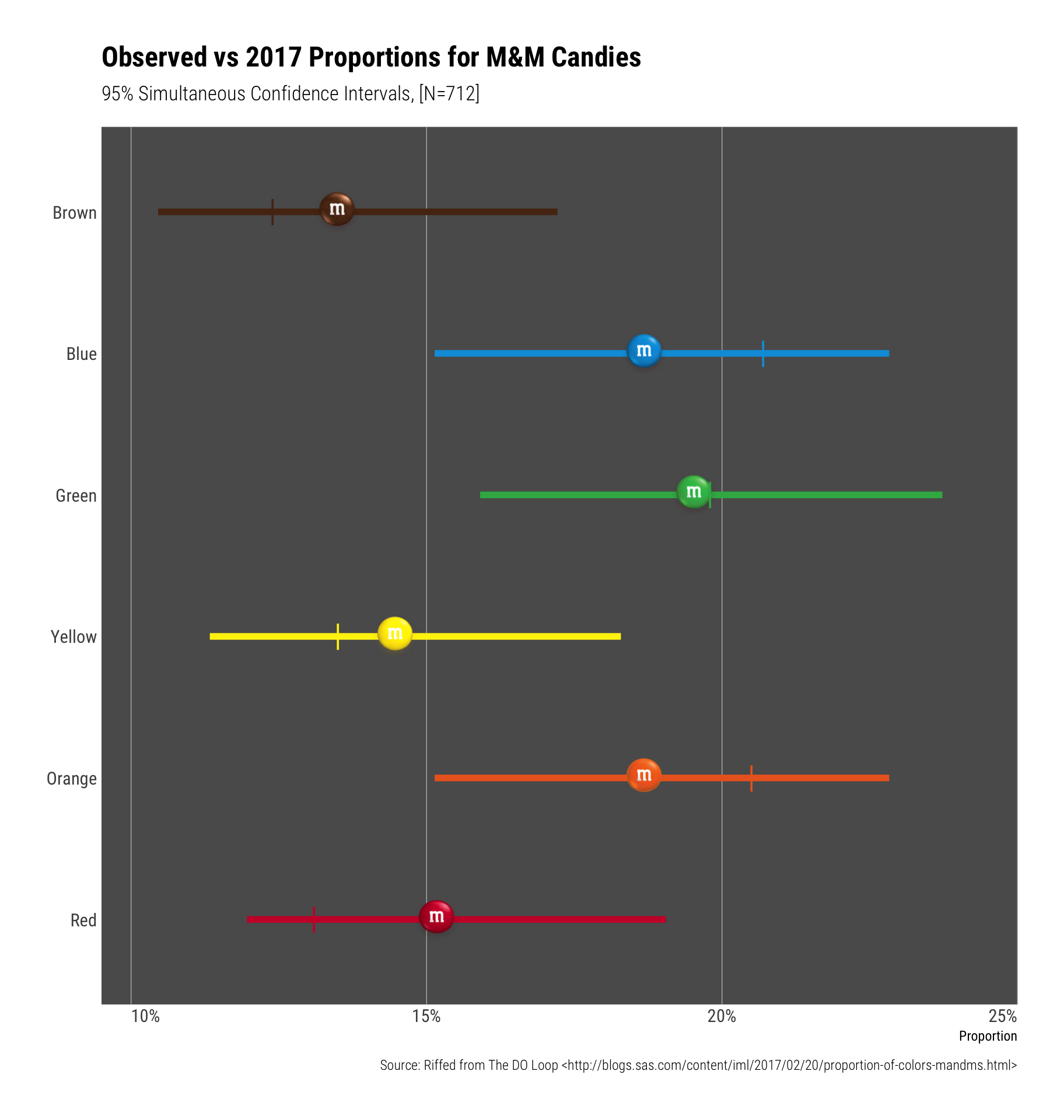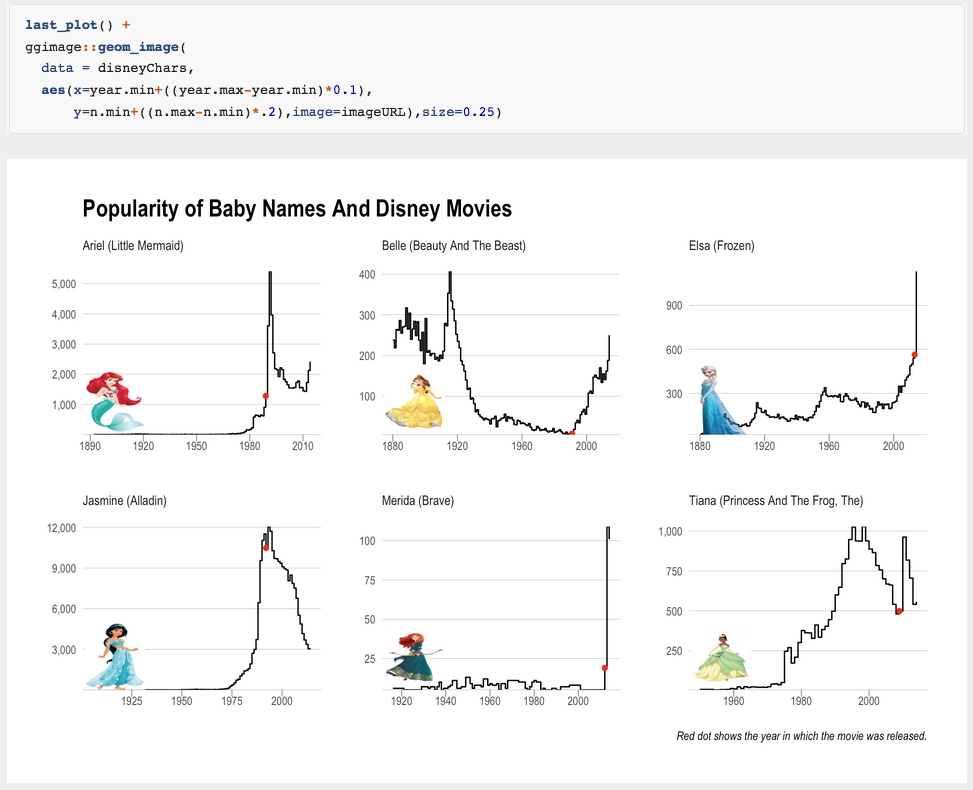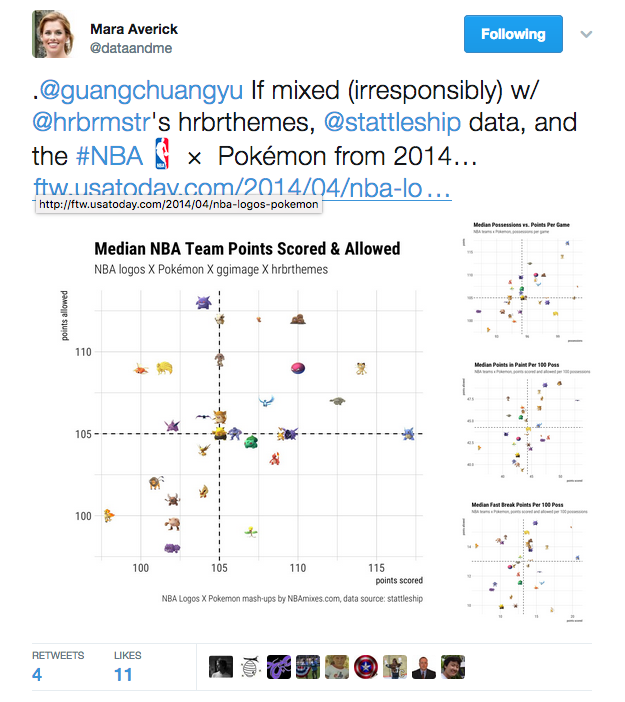ggimage是通用的包，所以可以被应用于不同的领域/场景中，起码可以让我们画出更好玩的图出来，后续我有时间的话，会写一个draw_key_image的函数，实现使用图片来当legend key的功能。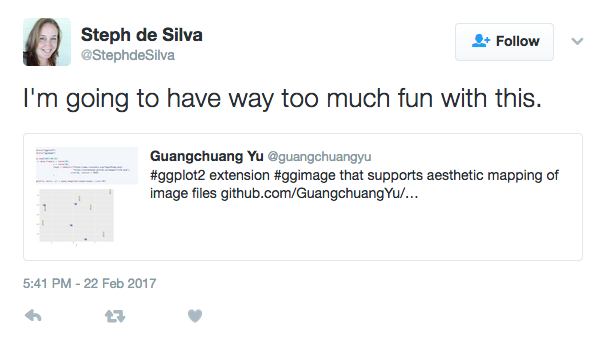## 参考资料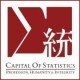## 《ggimage：ggplot2中愉快地使用图片》有1个想法

1.Maverick Chan说道：

感谢分享～